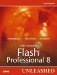# Sorting Arrays

Sorting plays an important role in using arrays. With sorting, you can put names in alphabetical order, put prices from greatest to least, and even see who has the highest score so far in a video game.

There are two types of sorting: One involves a general sort on the elements in an array, and the other involves the sorting of nested arrays.

Let's go over the general sort method. Just call this method like you would any other method, and it sorts somewhat alphabetically. Here's an example:

` var fName:Array = new Array("David","Shelley","Linda","Jonathan","Kim"); fName.sort(); trace(fName); //output: David,Jonathan,Kim,Linda,Shelley `

The sort worked fine. So why did I mention it will sort "somewhat" alphabetically? As you'll notice, all the strings in the fName array start with a capital letter. However, change the first letter in "David" to a lowercase d and see the results:

` var fName:Array = new Array("david","Shelley","Linda","Jonathan","Kim"); fName.sort(); trace(fName); //output: Jonathan,Kim,Linda,Shelley,david `

This time, "david" is moved to the back, even though it's the same name. The sort method does not recognize "david" as being the same as "David" because it doesn't look at the letters themselves; instead, it looks at their keycodes (discussed in Chapter 9), in which capital letters come before lowercase letters. There are solutions to this, however, and that is where the arguments to the sort method come in. You can pass arguments to control how the sort method will sort. There are three arguments you can pass:

• 1 for when A appears after B in the sorted sequence

• -1 for when A appears before B in the sorted sequence

• 0 if A is equal to B

Lets see what each does to our example we have been using.

` var fName:Array = new Array("david","Shelley","Linda","Jonathan","Kim"); fName.sort(1); trace(fName); fName.sort(-1); trace(fName); fName.sort(0); trace(fName); //output: david,Jonathan,Kim,Linda,Shelley //        david,Jonathan,Kim,Linda,Shelley //        Jonathan,Kim,Linda,Shelley,david `

In this example, we created an array filled with peoples first names, except one of them was not capitalized confusing the sort method. We passed it three different arguments to see which way we wanted it sorted.

NOTE

If you pass the sort method 0 as an argument, it is the same as leaving it blank.

#### The sortOn Method

The sortOn method is an extremely tricky method to use. This method sorts nested arrays by the value of a specific named element in each array. The syntax is similar to other methods covered so far, but in the parentheses, you put the named field you want to sort all the nested arrays by. Each of the nested arrays you want to sort must have that named field in it. Let's take a look at an example:

` var one:Array = new Array(); one.a = "a"; one.b = "b"; one.c = "c"; var two:Array = new Array(); two.a = "b"; two.b = "c"; two.c = "a"; var three:Array = new Array(); three.a = "c"; three.b = "a"; three.c = "b"; var my_array:Array = new Array(one,two,three) trace(my_array .a); my_array.sortOn("b"); trace(my_array .a); //output: a //        c `

In this example, we first created the three arrays we are going to put in our main array. In each of the nested arrays, we created three named array elements: one, two, and three. Then we set each of the three named elements to three different string literal letters: a, b, or c. Then, we placed each of these three arrays in my_array. After that, we traced the named element a in the first nested array of my_array. Then we ran the sort based on the named element b in all the nested arrays. After the sort, we traced my_array again based on the named element a in the first array element, and this time it was c. Therefore, the sort was successful.Macromedia Flash Professional 8 Unleashed
ISBN: 0672327619
EAN: 2147483647
Year: 2005
Pages: 319